Question

Write the source code for a 16-bit adder using the ‘+’ operator. Use the following information as a guide:

a. Use the names in the Adder Symbol/diagram above to name your block and its ports (all lower-case). For example dataa[15:0] signal name in VHDL would be dataa : in unsiged (15 downto 0);
b. All inputs and outputs should be declared as type UNSIGNED vs STD_LOGIC_VECTOR. c. Do not worry about rollover with this adder. This adder is already wide enough to account for all the values it will be adding together.

d. Make sure to include the library and package declarations at the beginning of the file. You will need the IEEE.NUMERIC_STD package. e. If you would prefer using a different text editor, please feel free to do so. Just make sure you save your VHDL file in the project directory.

bleow is the code

====================================

LIBRARY IEEE;
USE IEEE.STD_LOGIC_1164.ALL;
USE IEEE.NUMERIC_STD.ALL;

ARCHITECTURE stimulus OF adder_tb IS

-- Component declaration for adder block
PORT(
dataa, datab: IN UNSIGNED(15 DOWNTO 0);
sum: OUT UNSIGNED(15 DOWNTO 0)
);
END COMPONENT;

-- Signals to connect to DUT
SIGNAL dataa, datab, sum : UNSIGNED (15 DOWNTO 0);

BEGIN -- beginning of architecture body

-- instantiate unit under test (adder)
(dataa => dataa, datab => datab, sum => sum);

-- Assign values to "dataa" and "datab" to test adder block
dataa <= x"0008", x"0000" AFTER 20 NS, x"000A" AFTER 30 NS;
datab <= x"0005", x"0001" AFTER 20 NS, x"0005" AFTER 30 NS;

END ARCHITECTURE stimulus;

library ieee;
use ieee.std_logic_1164.all;
use ieee.numeric_std.all;

PORT(
dataa, datab: IN UNSIGNED(15 DOWNTO 0);
sum: OUT UNSIGNED(15 DOWNTO 0)
);

architecture arch of adder is

begin

sum <= dataa + datab;

end arch;

----------------------------------------------------

--Simulation on ModelSim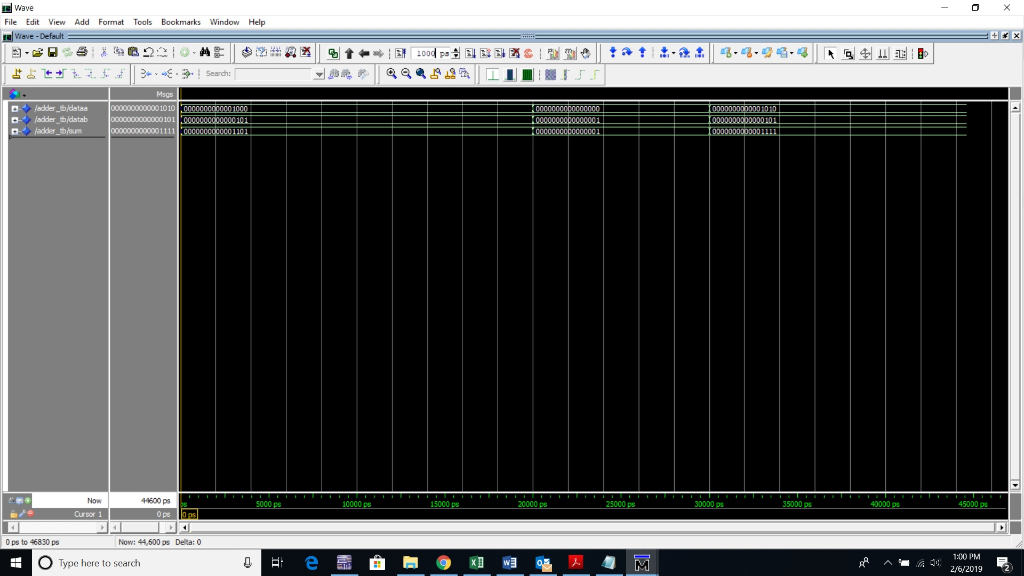#### Earn Coins

Coins can be redeemed for fabulous gifts.

Similar Homework Help Questions
• ### QUESTION 1 Complete the following peice of VHDL code with the necessary VHDL statements for a...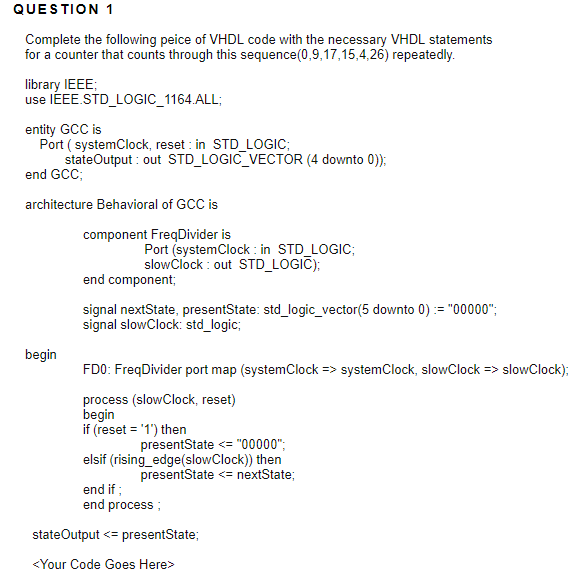QUESTION 1 Complete the following peice of VHDL code with the necessary VHDL statements for a counter that counts through this sequence(0,9,17,15,4,26) repeatedly. library IEEE use IEEE.STD_LOGIC_1164 ALL entity GCC is Port ( systemClock, reset in STD_LOGIC end GCC architecture Behavioral of GCC is stateOutput out STD LOGIC_ VECTOR (4 downto 0)) component FreqDivider is Port (systemClock in STD_LOGIC; slowClock: out STD LOGIC); end component, signal nextState, presentState: std_logic_vector(5 downto 0) := "00000"; signal slowClock: std_logic begin FD0: FreqDivider port...

• ### Write a test bench for the following VHDL code -------------------------------------------------------------------------------------------------------------------------------- LIBRARY ieee ; USE ieee.std_logic_1164.all ;...

Write a test bench for the following VHDL code -------------------------------------------------------------------------------------------------------------------------------- LIBRARY ieee ; USE ieee.std_logic_1164.all ; ENTITY registern IS GENERIC (N: INTEGER :=4);    -- INTEGER=32, 16, ….. PORT (D           : IN STD_LOGIC_VECTOR (N-1 downto 0); clk, reset, Load   : IN      STD_LOGIC ; Q           : OUT   STD_LOGIC_VECTOR (N-1 downto 0 )) ; END registern; ARCHITECTURE behavior OF registern IS BEGIN PROCESS (clk) BEGIN IF clk' EVENT AND clk='1' THEN IF (reset ='0') THEN    --synchronous reset Q<=(OTHERS=>’0’); ELSIF (L ='0') THEN Q<=D;...

• ### 8.(5 points).There is an error in following VHDL code. Find the error and correct (only that...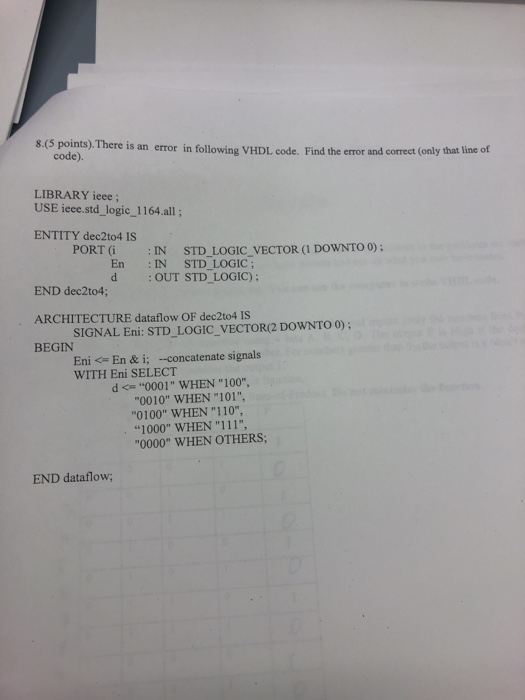8.(5 points).There is an error in following VHDL code. Find the error and correct (only that line of code). LIBRARY ieee; USE ieee.std_logic_1164.all; ENTITY dec2to4 IS PORT (i IN STD LOGIC VECTOR (1 DOWNTO 0); : En IN STD_LOGIC; d OUT STD LOGIC); END dec2to4; ARCHITECTURE dataflow OF dec2to4 IS BEGIN SIGNAL Eni: STD_LOGIC_VECTOR(2 DOWNTO 0); Eni <= En & i; -concatenate signals WITH Eni SELECT d <"0001" WHEN "100" "0010" WHEN "101", "0100" WHEN "110", "1000" WHEN "111", 0000"...

• ### 3. Study the VHDL code below for the multiplexer: -mux.vhd library ieee; use ieee.std_logic_1164.all; use ieee.std_logic_unsigned.all;...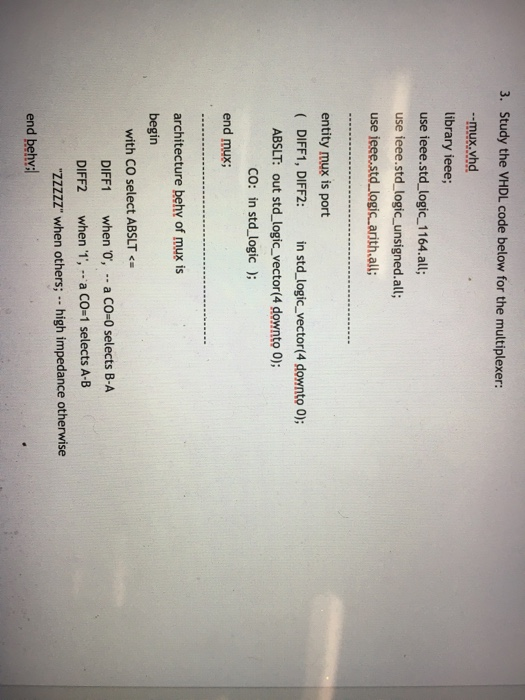3. Study the VHDL code below for the multiplexer: -mux.vhd library ieee; use ieee.std_logic_1164.all; use ieee.std_logic_unsigned.all; use jeee.std logic.arith.all; entity mux is port DIFF1, DIFF2: n std_logic_vector(4 downto O); ABSLT: out std_logic_vector(4 downto 0); CO: in std logic ); end mux; architecture behv of mux is begin with CO select ABSLT DIFF1 when o, a CO-0 selects B-A DIFF2 when 1',a Co-1 selects A-B "ZZZZZ" when others·.. high impedance otherwise end behy

• ### b. Suppose you are provided with a 4-bit ripple carry adder. It has the following entity...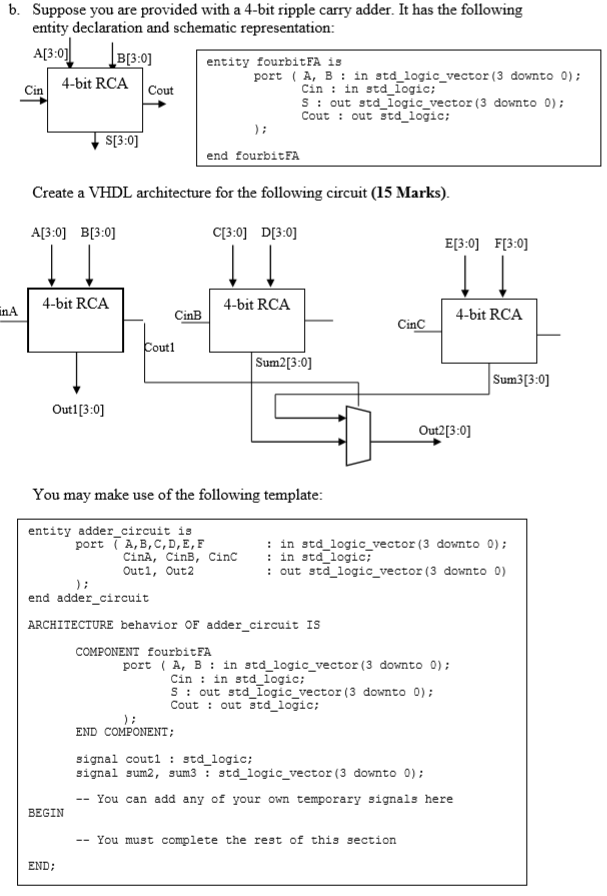b. Suppose you are provided with a 4-bit ripple carry adder. It has the following entity declaration and schematic representation А(3:0] B[3: entity fourbit FA is portA, B in std logic_vector (3 downto 0); 4-bit RCA Cin in std_logic; S out stdlogic_vector (3 downto 0) Cout out std logic: Cin Cout S3:0] end fourbitFA Create a VHDL architecture for the following circuit (15 Marks) C3:0] D[3:0] A[3:0] B[3:0] Е[3:0] F[3:0] 4-bit RCA 4-bit RCA inA 4-bit RCA CinB CinC Coutl...

• ### Draw the RTL schematic of the hardware that will be synthesized for the VHDL code below....

Draw the RTL schematic of the hardware that will be synthesized for the VHDL code below. entity unknown is port (x: in std_logic_vector(7 downto 0); op: in std_logic_vector(1 downto 0); clk: in std_logic; f: out std_logic_vector(7 downto 0)); end entity. architecture arch of unknown is signal a, b, c, d: std_logic_vector(7 downto 0); begin d <= x; process (clk) begin if (rising_edge(clk)) then a <= b; b <= c + a; c <= d; if (op = “00”) then f...

• ### Write a VHDL code using processes for the following logic circuit which include a shift register...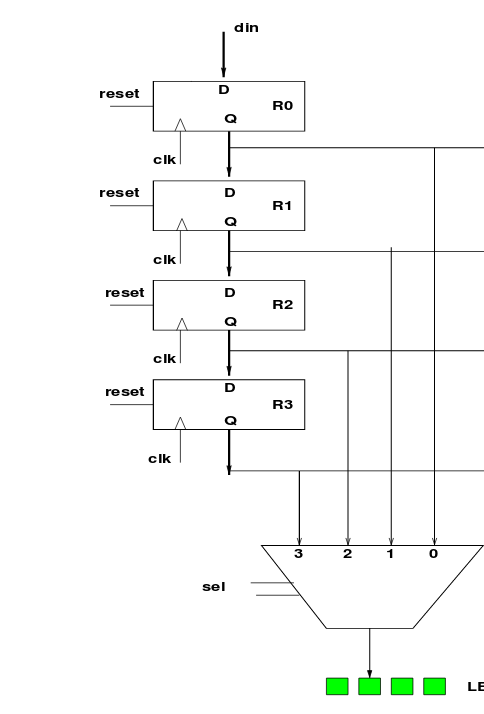Write a VHDL code using processes for the following logic circuit which include a shift register and 4x1 multiplexer. Use the entity below.              entity registers_min_max is port( din   : in std_logic_vector(3 downto 0);       reset : in std_logic;       clk   : in std_logic;       sel   : in std_logic_vector(1 downto 0);       reg_out : out std_logic_vector(3 downto 0)); end registers_min_max; din reset RO clk reset R1 A C clk reset R2 clk reset R3 clk 3 0 sel LE

• ### SRAP pr- Vivado 2017. Eile Edit Flow Iools Window Layout Yew HelpQuick Acces Ready VO Planning Fl...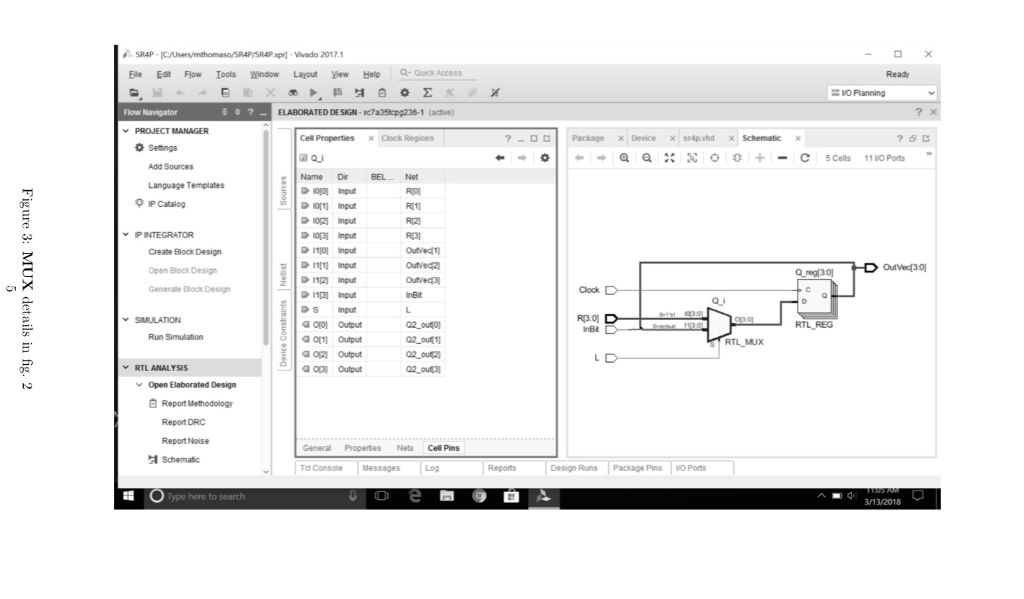SRAP pr- Vivado 2017. Eile Edit Flow Iools Window Layout Yew HelpQuick Acces Ready VO Planning Flow Navigator V PROJECT MANAGER Cell Properties x Clock Regions ?-OC Package x Device xsrapvhd × Schematic X O Setings Language Templates IP Catalog IPINTEGRATOR Open Block Design Qngl3이 OutVed3이 Generate Block Design SIMULATION R3.0 Run Simulation RTL ANALYSIS n Elaborated Design 白Report Methodology Report DRC Report Noise Schematic Td Console Messages Lg Reports Design Runs Package Pins VO Ports Type here to search...

• ### Some questions may require well bulum 1. HDL stands for? a. Hardware Design Language b. Hardware...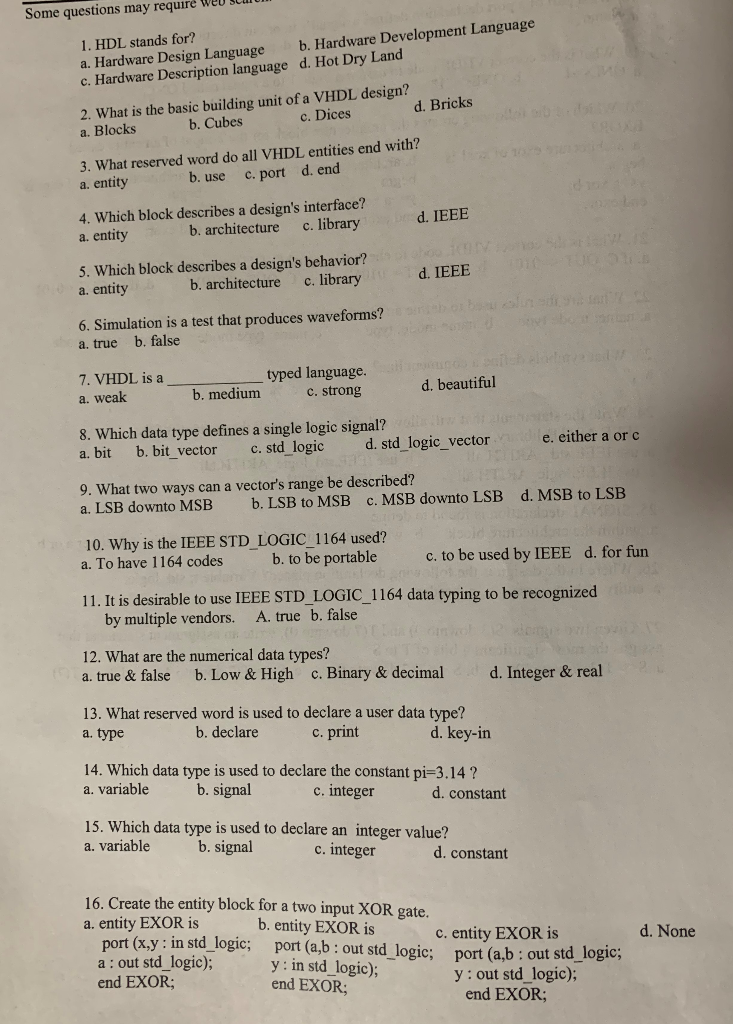Some questions may require well bulum 1. HDL stands for? a. Hardware Design Language b. Hardware Development Language c. Hardware Description language d. Hot Dry Land 2. What is the basic building unit of a VHDL design? a. Blocks b. Cubes c . Dices d. Bricks 3. What reserved word do all VHDL entities end with? a. entity b. use c. port d. end d. IEEE 4. Which block describes a design's interface? a. entity b. architecture c. library 5....

• ### car taillights help with code

x.øi5nt face="Times New Roman" size="3">I am trying to do a T-Bird tailight function using VHDL using model sim. I have been able to get the emergency (Hazard)and Left and right turn to work but can not get the brake light function to work. below is my codewhich turns on the hazard and turn signals correctly (on sparta s3 board or Model sim simulation)so what i want to do is "When the brakes are on, andwhen the hazard is on, it...